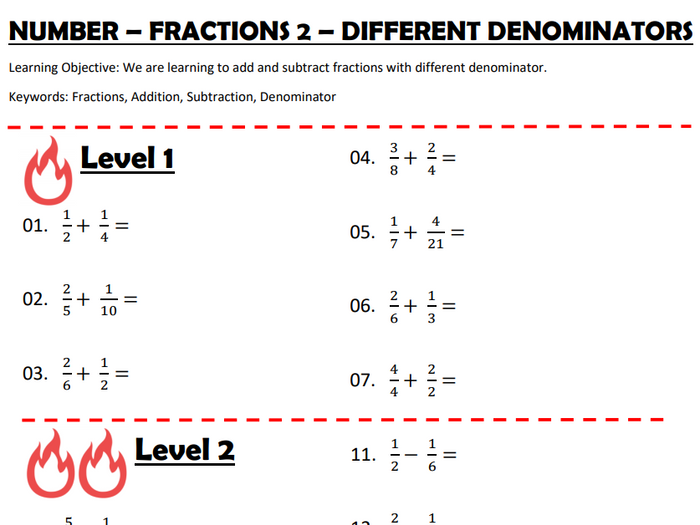Ideal revision tool for students wanting to recall and expand their knowledge on fractions. This worksheet focuses on ADDING and SUBTRACTING fractions with the DIFFERENT DENOMINATORS.

This worksheet focuses on simple conversion between fractions.

Level 1 - Addition of fractions with the different denominators (1-step only).

Level 2 - Subtraction of fractions with the different denominators (1-step only).

Level 3 - A bit of both (2-steps, 3-steps)

Level 4 - Answer given, form fractions with the different denominator to get the answer!

This worksheet is one of a five-part fraction pack.

\$1.88
Save for later

### Info

Created: Dec 8, 2019

pdf, 241 KB